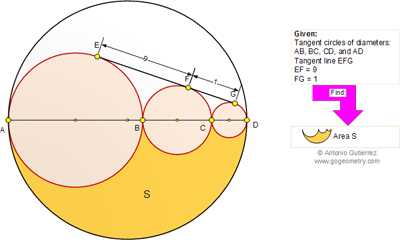## Saturday, May 7, 2011

### Geometry Problem 600: Circle, Area, Tangent, Semicircle, Tangency Point

Geometry Problem
Click the figure below to see the complete problem 600.1.Let r1= radius of circle dia. AB, r2= radius of circle dia. BC and r3=radius of circle dia. CD
Apply Pythagoras theorem to circles with radius r1 and r2 we get
(r1+r2)^2-(r1-r2)^2= 81 or 4.r1.r2=81
Similarly with circles r2 and r3
(r2+r3)^2-(r2-r3)^2=1 or 4.r2.r3=1
With circles r1 and r3
(r1+2.r2+r3)^2-(r1-r3)^2=100 or 4.r2^2+4.r1.r3=18

Solve system equations above we get r1=3/2 , r2= 27/2 , r3=1/6
Replace values of r1, r2 and r3 in following formula we will get the result
Area S= ½( pi.(r1+r2+r3)^2 – pi.r1^2-pi.r2^2-pi.r3^2))

Peter Tran

2.Minor typo correction
solution of system of equations give:
r1=27/2 ; r2=3/2 and r3=1/6

Peter Tran

3.Comment:
2S+π(r₁²+r₂²+r₃²)=π(r₁+r₂+r₃)²
S=π(r₁r₂+r₂r₃+r₃r₁)=π(81+1+100)/4=91π/2

4.Pravin,
Should the final answer not be 91π/4 ?
Ajit

5.Applying Problem 812.

Take a=9, b=1.

Then
S = π/4 (a^2 + b^2 + ab)
= π/4 (81 + 1 + 9)
= 91π/4

6.r1 --> Big circle
r2 --> medium circle
r3 --> small circle
r4 --> Biggest circle

Using formula of tangent of two circles we will have 3 equation:
1. (r1 + r2)^2 = (r1 - r2)^2 + 81
2. (r1 + r2 + r3)^2 = (r1 - r3)^2 + 100
3. (r2 + r3)^2 = (r2 - r3)^2 + 1

from the 1st equation: r1 . r2 = 81/4
from the 3rd equation: r2 . r3 = 1/4

r2 = 1/4r3

With the similarity of right angle triangle:
4. (r3 + r2)/(r1 + 2r2 + r3) = 1/10
5. (r2 - r3)/(r1 - r2) = 1/10

from the 4th equation and 5th equation:
9r3 + 8r2 = 10r2 - 9r3
9r3 = r2
9r3 = 1/4r3
r3 = 1/6
r2 = 3/2
r1 = 27/2
r4 = 27

Area of S = 91π/4

Thank u

7.y = radius of the smallest circle (circle on the right)
x = radius of the circle next to the smallest circle (circle on the middle)
r = radius of the circle on the left

By pythagorean theorem,
(r+x)² = (r-x)² + 81
rx = 81/4 or r = 81/4x (1)

(x+y)² = (x-y)² + 1
xy = 1/4 or y = 1/4x (2)

By similar triangles,

(x-y)/(r-x) = 1/9
9x - 9y = r-x (3)

Substitute (1) and (2) on (3), we get x = 3/2
then solve for r and y

the next is piece of cake